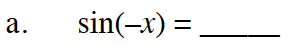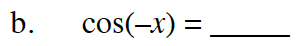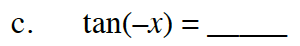### Home > PC3 > Chapter 8 > Lesson 8.3.2 > Problem8-100

8-100.
1. For each of the trigonometric functions below, decide if they are even, odd, or neither. Then use the even/odd properties of each function to complete each equation below. Homework Help ✎

1. sin(–x) = _____

2. cos(–x) = _____

3. tan(–x) = _____Review your work from parts (d) through (f) of problem 8-89.
An angle measure of 2πx = x in the unit circle.If sin(−x) = sin(x), then sine is an even function.
If sin(−x) = −sin(x), then sine is an odd function.Is the graph of y = cos(x) symmetric across the y-axis, or is it symmetric about the origin?Is the sign of tangent the same in Quadrants I and II or is the sign the same in Quadrants I and III?